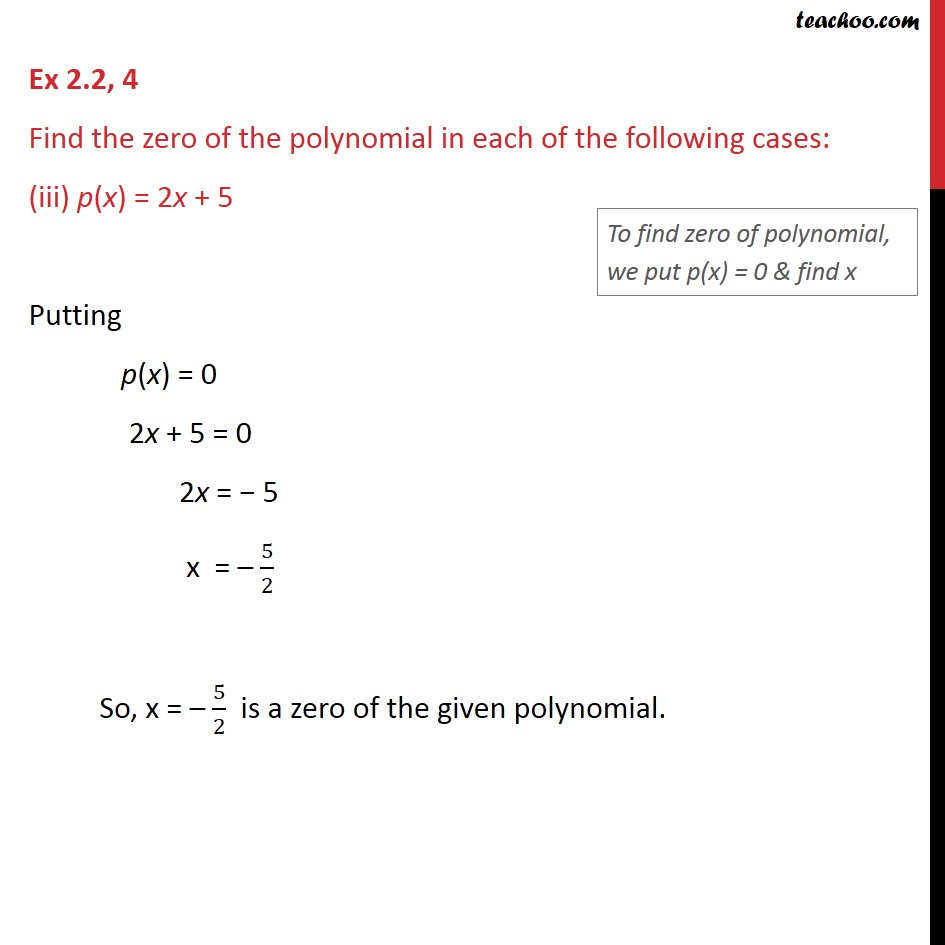Ex 2.2

Chapter 2 Class 9 Polynomials
Serial order wiseLearn in your speed, with individual attention - Teachoo Maths 1-on-1 Class

### Transcript

Ex 2.2, 4 Find the zero of the polynomial in each of the following cases: (iii) p(x) = 2x + 5 Putting p(x) = 0 2x + 5 = 0 2x = − 5 x = – 5/2 So, x = – 5/2 is a zero of the given polynomial.